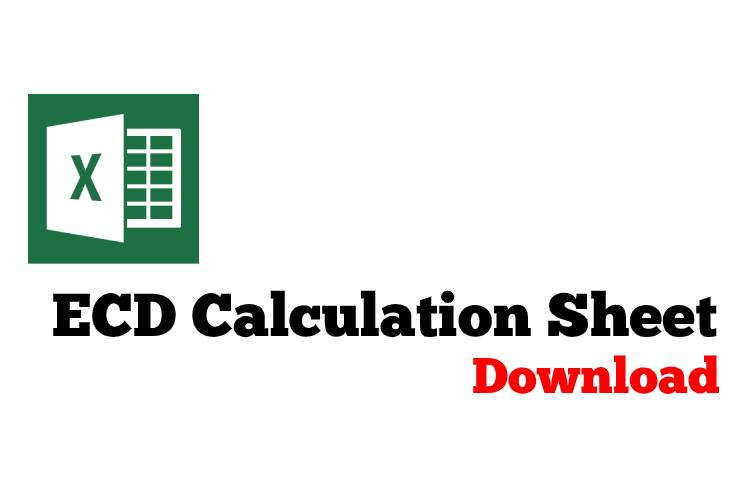## Pressure Loss and Equivalent Circulating Density Review

This example that I got from my junior member is very simple but it helps you a lot to understand about how to determine pressure loss during normal circulation.

Information given is listed below;Circulate at 3 bottom up through open end tubing (Down tubing and up annulus) with 12.7 ppg mud.

Pump pressure = 1000 psi

Annulus friction loss = 50 psi

Inside tubing friction loss = 925 psi

Surface line friction loss = 25 psi

## Calculate Equivalent Circulation Density (ECD) with complex engineering equations

These formulas below are used for complex calculation for annular pressure loss and equivalent circulating density. I think this calculation will give you more accurate result than a simple equation. Please follow the following steps how to calculate annular pressure loss and ECD.

1. Determine n: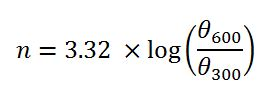2. Determine K: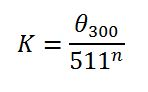3. Determine annular velocity (v) in ft/min: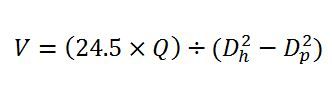4. Determine critical velocity (Vc) in ft/min: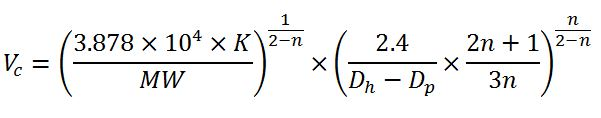5. Pressure loss for laminar flow (Ps), psi:6. Pressure loss for turbulent flow (Ps), psi:7. Determine equivalent circulating density (ECD), ppg: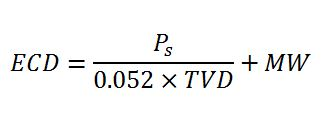Abbreviation meaning

θ300: viscometer dial reading at 300 rpm
θ600: viscometer dial reading at 600 rpm
Q: Flow rate in gpm
Dh: Diameter of hole
Dp: Diameter of drill pipe, drill collar or BHA in ft
v: annular velocity in ft/min
L: length of drill pipe, drill collar or BHA in ft
MW: Mud Weight
PV: Plastic viscosity

Example: Equivalent circulating density (ECD) in ppg by using following data:

Mud weight = 9.5 ppg
θ300 = 40
θ600 = 60
Plastic viscosity = 20 cps
Circulation rate = 650 gpm
Hole diameter = 8.5 in.
Drill collar OD = 6.75 in.
Drill pipe OD = 5.0 in
Drill collar length = 600 ft
Drill pipe length = 10,000 ft
True vertical depth = 9,000 ft

1. Determine n: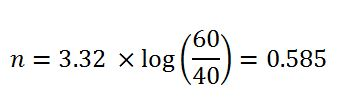2. Determine K: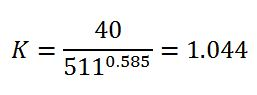3. Determine annular velocity (v) in ft/min around drill pipe: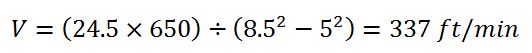4. Determine critical velocity (Vc) in ft/min around drill pipe: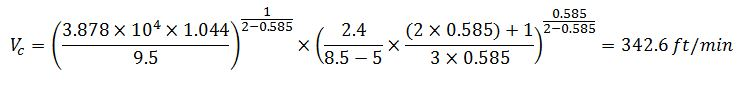The annular velocity around drill pipe is less than the critical velocity around drill pipe so this is laminar flow. The equation #5 (for laminar flow) must be applied in this case.

Pressure loss for turbulent flow (Ps), psi:5. Determine annular velocity (v) in ft/min around drill collar: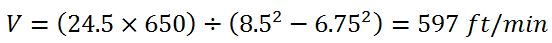6. Determine critical velocity (Vc) in ft/min around drill collar: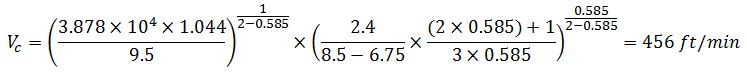The annular velocity around drill collar is more than the critical velocity around drill collar so this is turbulent flow. The equation #6 (for turbulent flow) must be applied in this case.

Pressure loss for laminar flow (Ps), psi:Total annular pressure loss = annular pressure loss around drill pipe + annular pressure loss around drill collar

Ps=271.3+81.5 = 352.8psi

7. Determine equivalent circulating density (ECD), ppg:Please find the Excel sheet for calculating ECD (engineering calculation)

Ref books: Lapeyrouse, N.J., 2002. Formulas and calculations for drilling, production and workover, Boston: Gulf Professional publishing.

Bourgoyne, A.J.T., Chenevert , M.E. & Millheim, K.K., 1986. SPE Textbook Series, Volume 2: Applied Drilling Engineering, Society of Petroleum Engineers.

Mitchell, R.F., Miska, S. & Aadny, B.S., 2011. Fundamentals of drilling engineering, Richardson, TX: Society of Petroleum Engineers.

## Equivalent Circulating Density (ECD) in both Oilfield and Metric UnitEquivalent Circulating Density (ECD) is the effective density that combines current mud density and annular pressure drop. ECD is vital for drilling engineering since it limits how depth of each section to be drilled and leads to losses.The equivalent circulating density formula is shown below;

## Oilfield Unit

Equivalent Circulating Density (ECD) = (Annular Pressure Loss ÷ 0.052 ÷ True Vertical Depth (TVD)) + (Current Mud Weight)

Equivalent Circulating Density (ECD) in ppg

Annular Pressure Loss in psi

True Vertical Depth (TVD) in ft

Current Mud Weight in pppg

Example:

Annular pressure loss = 400 psi
True Vertical Depth = 8,000 ft
Current mud weight in ppg = 10 ppg

ECD in ppg =   (400 psi ÷ 0.052 ÷ 8,000 ft) +10.0 ppg

ECD = 11.0 ppg

## Metric Unit

Equivalent Circulating Density (ECD) = (Annular Pressure Loss × 1000 ÷ 9.81÷ True Vertical Depth (TVD) ) + (Current Mud Weight)

Equivalent Circulating Density (ECD) in kg/m3

Annular Pressure Loss in KPa

True Vertical Depth (TVD) in m

Current Mud Weight in kg/m3

Example:

Annular pressure loss = 2760 KPa
True Vertical Depth = 2,440 m
Current mud weight in ppg = 1200  kg/m3

ECD in ppg =  (2,760 Kpa  × 1000 ÷ 9.81÷ 2,440 m )  + 1,200 kg/m3

ECD = 1,315 kg/m3

Please find the Excel sheet to calculate Equivalent Circulating Density (ECD)